# Roku SDK Documentation : Animation Markup

Node Class References: Animation, SequentialAnimation, ParallelAnimation
Interpolator Node References: Vector2DFieldInterpolator, FloatFieldInterpolator, ColorFieldInterpolator

The following XML SceneGraph markup examples demonstrate how to animate renderable nodes. This allows you to move text, shapes, pictures, and videos around the display screen, fade them in and/or out of view, slide them completely off the display screen area, move them over each other, and change their color.

All of these animation effects are accomplished using Animation node classes that target specific fields of renderable nodes, changing them over a specified time to achieve the animation effect. Depending on the fields to animate, you will use one of three interpolator node classes:

• Vector2DFieldInterpolator
• FloatFieldInterpolator
• ColorFieldInterpolator

Let's look at how to use each of the interpolator node classes to animate renderable nodes. Then let's look at the SequentialAnimation and ParallelAnimation nodes, which allow you to run several animations either sequentially or in parallel.

## Vector2DFieldInterpolator Markup

Example Application: AnimationV2DExample.zip
Interpolator Node Reference: Vector2DFieldInterpolator

In this example, we'll use a Vector2DFieldInterpolator node to animate the `translation` field of a Rectangle node, causing it to move around the screen. If you look at the `animationv2dscene.xml` file in the example application package, you'll note we are using a similar Rectangle node definition to some we used in previous tutorial examples, specifically the big blue rectangle in Z-Order/Parent-Child Markup:

`<Rectangle `
`  id = "movingRectangle" `
`  width = "720" `
`  height = "240" `
`  color = "0x1010EBFF" />`

But we're defining it as a child node of a larger Rectangle node to set the boundaries of the animation. We make this parent Rectangle node invisible by setting the alpha channel to 0x00:

Vector 2D Animation Example
```<children>

<Rectangle
id = "exampleRectangle"
width = "900"
height = "330"
color = "0x10101000" >

<Rectangle
id = "movingRectangle"
width = "720"
height = "240"
color = "0x1010EBFF" />

</Rectangle>

<Animation
id = "exampleVector2DAnimation"
duration = "5"
easeFunction = "linear" >

<Vector2DFieldInterpolator
key = "[ 0.0, 0.5, 1.0 ]"
keyValue = "[ [0.0,0.0], [180.0,90.0], [0.0,0.0] ]"
fieldToInterp = "movingRectangle.translation" />

</Animation>

</children>```

We're actually only using this parent Rectangle node to establish the dimension limits of the animation; it is not visible in the example, but allows us to center the moving rectangle at the mid-point of the animation.

This all might look a little confusing so let's break it down piece by piece:

• We added an ID for the Animation node to target the node by BrightScript code in the `init()` function:

` id = "exampleVector2DAnimation" `

• We set the duration of the animation to five seconds:

` duration = "5" `

• We are using the most typical "linear" definition of the `easeFunction` field, which is actually the default animation ease function:

` easeFunction = "linear" `

• In the Vector2DFieldInterpolator child node, we have two fields with arrays of values that must have the same number of values:

` key = "[ 0.0, 0.5, 1.0 ]" ` `keyValue = "[ [0.0,0.0], [180.0,90.0], [0.0,0.0] ]" `

• the `key` field array contains the fractional values of the five second duration of the animation where the field value specified by the corresponding `keyValue` array is set; for this animation there are three key values which basically divide the animation into three parts, a beginning (0.0), a middle (0.5), and an end (1.0)

• the `keyValue` field array contains the values of the Rectangle node `translation` field that will be set at the corresponding fractional value in the `key` array, to position the rectangle at the specified X,Y position on the display screen

• The `fieldToInterp` field then defines which of the Rectangle node fields to animate; in this case it is the `translation` field of the `movingRectangle` node as identified by its ID field:

` fieldToInterp = "movingRectangle.translation" `

Now to start the animation, we have to set the `control` field of the Animation node object to `play` using BrightScript in the `init()` function, where we also set the `repeat` field to true to have the animation to repeat (endlessly) after completing:

`m.vector2danimation = m.top.FindNode("exampleVector2DAnimation")`
`m.vector2danimation.repeat = true`
`m.vector2danimation.control = "start"`

The following shows the position of the text on the display screen correlated with the `duration` field time, the `key` field fractional portion of the animation, and the `keyValue` position of the moving rectangle: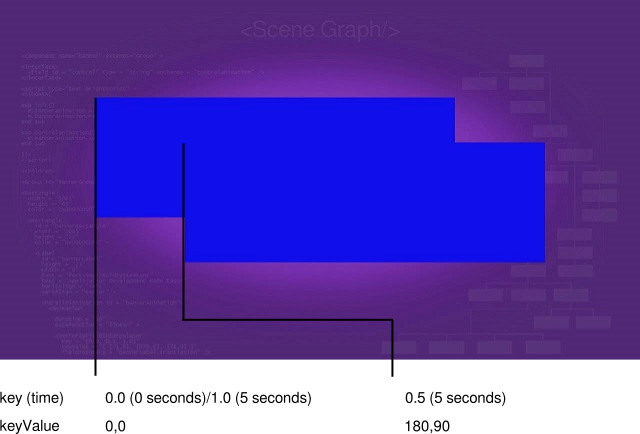It can take a little while to correlate the definitions of these fields with the movement of the rectangle on the screen, so take some time and watch it move back and forth...and back and forth...

## FloatFieldInterpolator Markup

Example Application: AnimationFloatExample.zip
Interpolator Node Reference: FloatFieldInterpolator

In this example, we'll use a FloatFieldInterpolator node to animate the `opacity` field of a Poster node, causing it to fade in and out of visibility. We'll go ahead and use the same Poster node definition we used in Renderable Node Markup previously. The following shows the XML Animation node and FloatFieldInterpolator child node definitions for this:

`<Animation `
`  id = "exampleFloatAnimation" `
`  duration = "5" `
`  easeFunction = "linear" >`

`  <FloatFieldInterpolator `
`    key = "[ 0.0, 0.5, 1.0 ]" `
`    keyValue = "[ 1.0, 0.0, 1.0 ]" `
`    fieldToInterp = "examplePoster.opacity" />`

`</Animation>`

We've kept this example simple by using the same `duration` and `key` field definitions as in the previous Vector2DFieldInterpolator example. This means that the animation will again last five seconds, and be divided into three parts: a beginning at 0 seconds (`key` value 0.0), a middle at 2.5 seconds (`key` value 0.5), and an end at five seconds (`key` value 1.0). Note that this causes the `examplePoster` to gradually disappear from the display screen, be fully invisible at 2.5 seconds, then gradually reappear until it is completely visible at five seconds. And again, because the animation `repeat` field was set to `true`, this happens over and over and over again...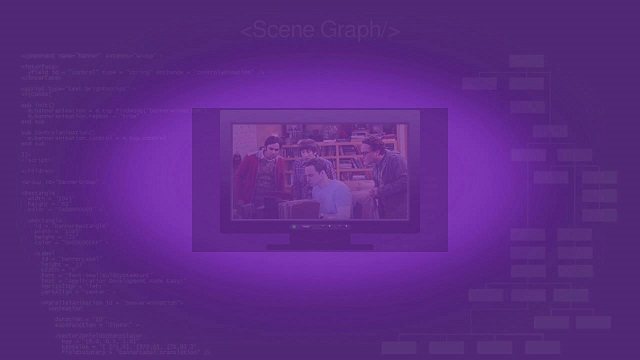## ColorFieldInterpolator Markup

Example Application: AnimationColorExample.zip
Interpolator Node Reference: ColorFieldInterpolator

For this final Animation node interpolator example, we'll change the color of a rectangle. To do this, we must use the ColorFieldInterpolator node, which can change the `color` field of a target node over the time of the animation specified in the Animation node `duration` field. Once again, to keep it simple, we'll use the same `duration`, and `key` field definitions used in the previous interpolator examples, and use the same Rectangle node markup from Renderable Node Markup:

`<Animation `
`  id = "exampleColorAnimation" `
`  duration = "5" `
`  easeFunction = "linear" >`

`  <ColorFieldInterpolator `
`    key = "[ 0.0, 0.5, 1.0 ]" `
`    keyValue = "[ 0x1010EBFF, 0x10101FFF, 0x1010EBFF ]" `
`    fieldToInterp = "exampleRectangle.color" />`

`</Animation>`

Because we used many of the same field definitions as the previous interpolator examples, it should be clear that the only difference here is that the animation changes the `color` field of the target `exampleRectangle` rectangle, so it changes the rectangle color rather than moving or fading it. The color changes from blue (0x1010EBFF) at 0 seconds, to black (actually a very dark shade of blue, 0x10101FFF) at 2.5 seconds, and back to blue at five seconds.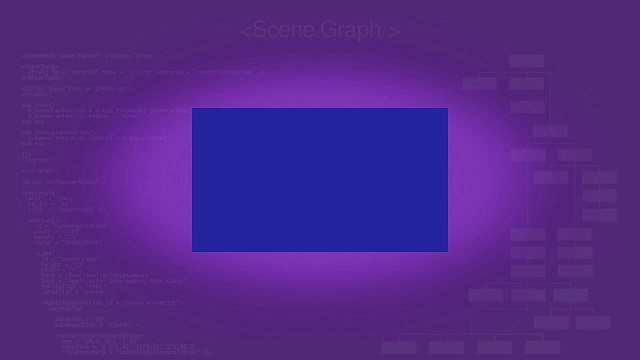## SequentialAnimation Markup

Example Application: AnimationSequentialExample.zip
Node Class Reference: SequentialAnimation

The SequentialAnimation node class allows you to group several related animations together to run in sequence, one after another.

Remember how we used the big blue rectangle from previous tutorial examples in Vector2DFieldInterpolator Markup? That rectangle was one of three we used to show how Roku SceneGraph implements z-order and parent-child node relationships in Z-Order/Parent-Child Markup. `AnimationSequentialExample.zip` shows how to move those rectangles so that you see each underlying rectangle in sequence.

Sequential Animation Example
```<children>

<Group id = "animatedRectangles" >

<Rectangle
id = "lowestRectangle"
width = "180"
height = "60"
color = "0xEB1010FF" />

<Rectangle
id = "middleRectangle"
width = "360"
height = "120"
color = "0x10EB10FF" />

<Rectangle
id = "highestRectangle"
translation = "[ 180.0, 90.0 ]"
width = "720"
height = "240"
color = "0x1010EBFF" />

<SequentialAnimation id = "exampleSequentialAnimation" >

<Animation
duration = "3"
easeFunction = "linear" >

<Vector2DFieldInterpolator
key = "[ 0.0, 1.0 ]"
keyValue = "[ [0.0,0.0], [180.0,90.0] ]"
fieldToInterp = "highestRectangle.translation" />

</Animation>

<Animation
duration = "3"
easeFunction = "linear" >

<Vector2DFieldInterpolator
key = "[ 0.0, 1.0 ]"
keyValue = "[ [0.0,0.0], [60.0,30.0] ]"
fieldToInterp = "middleRectangle.translation" />

</Animation>

<Animation
duration = "3"
easeFunction = "linear" >

<Vector2DFieldInterpolator
key = "[ 0.0, 1.0 ]"
keyValue = "[ [60.0,30.0], [0.0,0.0] ]"
fieldToInterp = "middleRectangle.translation" />

</Animation>

<Animation
duration = "3"
easeFunction = "linear" >

<Vector2DFieldInterpolator
key = "[ 0.0, 1.0 ]"
keyValue = "[ [180.0,90.0], [0.0,0.0] ]"
fieldToInterp = "highestRectangle.translation" />

</Animation>

</SequentialAnimation>

</Group>

</children>```

The first step is to define the rectangles, which we've done before. In this case though, we don't define the `translation` field values for the rectangles, except for the last rectangle, since we'll be moving the rectangles by changing the values of the `translation` fields in the animations. But we do set the `translation` field value of the last rectangle, the big blue rectangle, to set the boundary of the animation at its furthest point. This allows us to center the animated rectangles as we've done in all the tutorial examples.

Then we define the `SequentialAnimation` node, which will be the parent node of several child animation nodes that move the rectangles in sequence. We define the parent SequentialAnimation node ID as `exampleSequentialAnimation`, so that we can run all the child animation nodes in sequence by setting the `control` field value of this parent node to `start` in the `init()` function. After that, we define the several child animation nodes, to be run in the sequence of their definition. Note that each child animation node is a complete animation with a `duration` field, and a child interpolator node. For example, the first child animation node moves the big blue rectangle from X,Y coordinates 0,0 to 180,90 in three seconds:

`<Animation `
`  duration = "3" `
`  easeFunction = "linear" >`

`  <Vector2DFieldInterpolator `
`    key = "[ 0.0, 1.0 ]" `
`    keyValue = "[ [0.0,0.0], [180.0,90.0] ]" `
`    fieldToInterp = "highestRectangle.translation" />`

`</Animation>`

And each successive child animation nodes moves one of the rectangles in a similar manner.

We start the animations in the `init()` function in the usual way, by setting the `control` field value of the parent SequentialAnimation node to `start`:

`m.examplesequentialanimation = m.top.findNode("exampleSequentialAnimation")`
`m.examplesequentialanimation.repeat = true`
`m.examplesequentialanimation.control = "start"`

And the result is: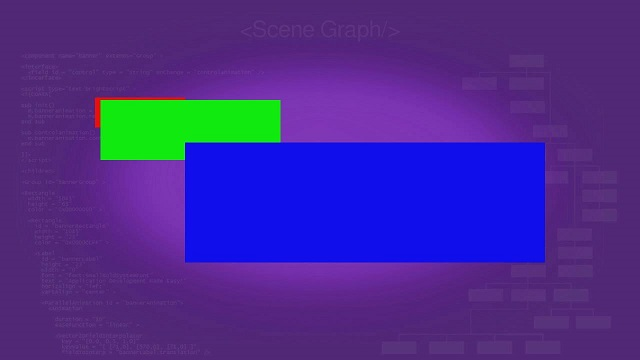## ParallelAnimation Markup

Example Application: AnimationParallelExample.zip
Node Class Reference: ParallelAnimation

The ParallelAnimation node class is very similar to the SequentialAnimation node class, in that it is a parent node to several child animation nodes. But instead of running each child animation node in sequence, the child animation nodes are run at the same time, in parallel.

Let's animate the same three rectangles in SequentialAnimation Markup to move the same way, but this time in parallel:

Parallel Animation Example
```<children>

<Group id = "animatedRectangles" >

<Rectangle
id = "lowestRectangle"
width = "180"
height = "60"
color = "0xEB1010FF" />

<Rectangle
id = "middleRectangle"
width = "360"
height = "120"
color = "0x10EB10FF" />

<Rectangle
id = "highestRectangle"
translation = "[ 180.0, 90.0 ]"
width = "720"
height = "240"
color = "0x1010EBFF" />

<ParallelAnimation id = "exampleParallelAnimation" >

<Animation
duration = "5"
easeFunction = "linear" >

<Vector2DFieldInterpolator
key = "[0.0, 0.5, 1.0]"
keyValue = "[ [0.0,0.0], [60.0,30.0], [0.0,0.0] ]"
fieldToInterp = "middleRectangle.translation" />

</Animation>

<Animation
duration = "5"
easeFunction = "linear" >

<Vector2DFieldInterpolator
key = "[0.0, 0.5, 1.0]"
keyValue = "[ [0.0,0.0], [180.0,90.0], [0.0,0.0] ]"
fieldToInterp = "highestRectangle.translation" />

</Animation>

</ParallelAnimation>

</Group >

</children>```

Again we set the boundary of the animation at its furthest point by setting the `translation` field of the big blue rectangle. And again we define a parent ParallelAnimation node with several child animation nodes. The child animation nodes differ from `AnimationSequentialExample.zip` in that they have an entire set of `keyValue` field values, to move the rectangles to their furthermost position, then back to their starting position.

And again the parallel animation is started in the `init()` function:

`m.exampleparallelanimation = m.top.FindNode("exampleParallelAnimation")`
`m.exampleparallelanimation.repeat = true`
`m.exampleparallelanimation.control = "start"`

And the result is: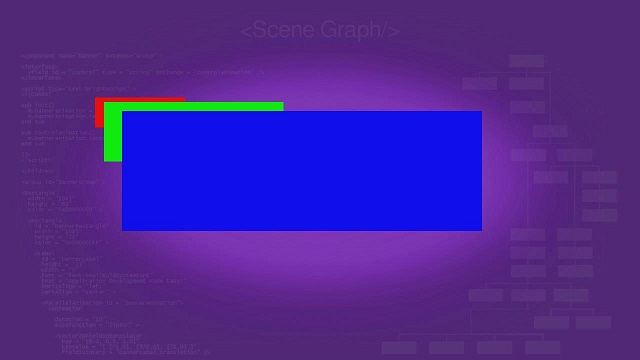## Attachments: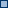label800.jpg (application/octet-stream)labelscroll.jpg (application/octet-stream)PosterAnimation.zip (application/octet-stream)LabelAnimation.zip (application/octet-stream)RectangleAnimation.zip (application/octet-stream)LabelAnimation.zip (application/zip)PosterAnimation.zip (application/zip)RectangleAnimation.zip (application/zip)labelanimation.jpg (image/jpeg)labelanimation.jpg (image/jpeg)AnimationV2DExample.zip (application/zip)animv2ddoc.jpg (image/jpeg)AnimationFloatExample.zip (application/zip)animfloatdoc.jpg (image/jpeg)animcolordoc.jpg (image/jpeg)AnimationSequentialExample.zip (application/zip)animsequencedoc.jpg (image/jpeg)AnimationParallelExample.zip (application/zip)animparalleldoc.jpg (image/jpeg)AnimationV2DExample.zip (application/zip)AnimationFloatExample.zip (application/zip)AnimationColorExample.zip (application/zip)AnimationSequentialExample.zip (application/zip)AnimationParallelExample.zip (application/zip)animv2ddoc.jpg (image/jpeg)animv2ddoc14pt.jpg (image/jpeg)animfloatdoc.jpg (image/jpeg)animcolordoc.jpg (image/jpeg)animsequencedoc.jpg (image/jpeg)animparalleldoc.jpg (image/jpeg)